# US9568320B2 - Method and apparatus for estimation of center of gravity using accelerometers - Google Patents

Method and apparatus for estimation of center of gravity using accelerometers Download PDF

## Info

Publication number
US9568320B2
US9568320B2 US14/704,650 US201514704650A US9568320B2 US 9568320 B2 US9568320 B2 US 9568320B2 US 201514704650 A US201514704650 A US 201514704650A US 9568320 B2 US9568320 B2 US 9568320B2
Authority
US
United States
Prior art keywords
ω
right arrow
arrow over
accelerometers
over
Prior art date
Legal status (The legal status is an assumption and is not a legal conclusion. Google has not performed a legal analysis and makes no representation as to the accuracy of the status listed.)
Active, expires
Application number
US14/704,650
Other versions
US20160327394A1 (en
Inventor
Moustafa Elshafei Ahmed Elshafei
Current Assignee (The listed assignees may be inaccurate. Google has not performed a legal analysis and makes no representation or warranty as to the accuracy of the list.)
King Fahd University of Petroleum and Minerals
Original Assignee
King Fahd University of Petroleum and Minerals
Priority date (The priority date is an assumption and is not a legal conclusion. Google has not performed a legal analysis and makes no representation as to the accuracy of the date listed.)
Filing date
Publication date
Application filed by King Fahd University of Petroleum and Minerals filed Critical King Fahd University of Petroleum and Minerals
Priority to US14/704,650 priority Critical patent/US9568320B2/en
Assigned to KING FAHD UNIVERSITY OF PETROLEUM AND MINERALS reassignment KING FAHD UNIVERSITY OF PETROLEUM AND MINERALS ASSIGNMENT OF ASSIGNORS INTEREST (SEE DOCUMENT FOR DETAILS). Assignors: AL-RAWASHDEH, YAZAN MOHAMMAD, ELSHAFEI, MOUSTAFA ELSHAFEI AHMED, AL-MALKI, MOHAMMAD FAHD
Publication of US20160327394A1 publication Critical patent/US20160327394A1/en
Application granted granted Critical
Publication of US9568320B2 publication Critical patent/US9568320B2/en
Application status is Active legal-status Critical

• 230000001133 acceleration Effects 0 claims description 56
• 238000005259 measurements Methods 0 claims description 49
• 238000000034 methods Methods 0 claims description 16
• 238000004364 calculation methods Methods 0 claims description 11
• 239000011159 matrix materials Substances 0 claims description 10
• 239000003570 air Substances 0 claims description 7
• 239000010432 diamond Substances 0 claims description 5
• 238000001914 filtration Methods 0 claims description 3
• 239000000203 mixtures Substances 0 claims description 3
• 230000015654 memory Effects 0 description 8
• 238000000354 decomposition Methods 0 description 6
• 239000002609 media Substances 0 description 6
• 238000003860 storage Methods 0 description 6
• 230000000694 effects Effects 0 description 4
• 239000000047 products Substances 0 description 3
• 238000004513 sizing Methods 0 description 3
• 230000001413 cellular Effects 0 description 2
• 238000004891 communication Methods 0 description 2
• 230000000875 corresponding Effects 0 description 2
• 230000001419 dependent Effects 0 description 2
• 229910003460 diamond Inorganic materials 0 description 2
• 230000014509 gene expression Effects 0 description 2
• 230000001965 increased Effects 0 description 2
• 230000002093 peripheral Effects 0 description 2
• 238000005070 sampling Methods 0 description 2
• 230000035945 sensitivity Effects 0 description 2
• 206010034719 Personality change Diseases 0 description 1
• 230000003044 adaptive Effects 0 description 1
• 230000000996 additive Effects 0 description 1
• 239000000654 additives Substances 0 description 1
• 239000011162 core materials Substances 0 description 1
• 230000001808 coupling Effects 0 description 1
• 238000005225 electronics Methods 0 description 1
• 238000005516 engineering processes Methods 0 description 1
• 230000002708 enhancing Effects 0 description 1
• 238000000802 evaporation-induced self-assembly Methods 0 description 1
• 230000010006 flight Effects 0 description 1
• 239000000446 fuel Substances 0 description 1
• 230000036541 health Effects 0 description 1
• 230000001976 improved Effects 0 description 1
• 238000009434 installation Methods 0 description 1
• 238000005305 interferometry Methods 0 description 1
• 230000000670 limiting Effects 0 description 1
• 230000004048 modification Effects 0 description 1
• 238000006011 modification Methods 0 description 1
• 230000003287 optical Effects 0 description 1
• 239000004626 polylactic acid Substances 0 description 1
• 230000002829 reduced Effects 0 description 1
• 230000001603 reducing Effects 0 description 1
• 230000004044 response Effects 0 description 1
• 239000010936 titanium Substances 0 description 1
• 229910052719 titanium Inorganic materials 0 description 1
• 229910001868 water Inorganic materials 0 description 1
• 229910052724 xenon Inorganic materials 0 description 1

## Images

•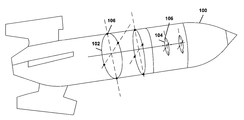•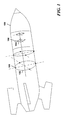•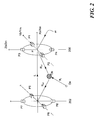•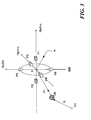•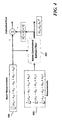•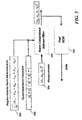•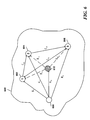•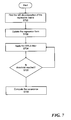•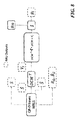•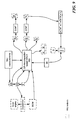•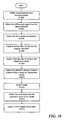•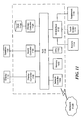•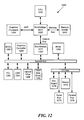•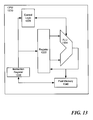## Classifications

• GPHYSICS
• G01MEASURING; TESTING
• G01CMEASURING DISTANCES, LEVELS OR BEARINGS; SURVEYING; NAVIGATION; GYROSCOPIC INSTRUMENTS; PHOTOGRAMMETRY OR VIDEOGRAMMETRY
• G01C21/10Navigation; Navigational instruments not provided for in preceding groups G01C1/00-G01C19/00 by using measurements of speed or acceleration
• G01C21/12Navigation; Navigational instruments not provided for in preceding groups G01C1/00-G01C19/00 by using measurements of speed or acceleration executed aboard the object being navigated; Dead reckoning
• G01C21/16Navigation; Navigational instruments not provided for in preceding groups G01C1/00-G01C19/00 by using measurements of speed or acceleration executed aboard the object being navigated; Dead reckoning by integrating acceleration or speed, i.e. inertial navigation
• GPHYSICS
• G01MEASURING; TESTING
• G01MTESTING STATIC OR DYNAMIC BALANCE OF MACHINES OR STRUCTURES; TESTING OF STRUCTURES OR APPARATUS, NOT OTHERWISE PROVIDED FOR
• G01M1/00Testing static or dynamic balance of machines or structures
• G01M1/12Static balancing; Determining position of centre of gravity
• G01M1/122Determining position of centre of gravity
• G01M1/125Determining position of centre of gravity of aircraft
• G01M1/127Determining position of centre of gravity of aircraft during the flight

## Abstract

A system and associated methodology determine navigation parameters of a vehicle under varying center of gravity position and varying unknown gravitational forces. The system uses high precision accelerometers arranged in a plurality of configurations.

## Description

BACKGROUND

Dynamic equations of an aircraft vehicle are derived under the assumption of a known and stationary Center of Gravity (CoG). Variations in loads result in a change in both of the aircraft vehicle's mass and the CoG position. The change in the CoG introduces undesirable couplings in flights dynamics. The variations in loads may be due to fuel consumption or change in payload of the aircraft vehicle. Knowledge of the CoG position is important for determination of the aircraft vehicle attitude, calculation of the various aerodynamics forces and torques, selection of the proper control strategy, and ensuring the aircraft vehicle stability.

An apparatus for tracking the center of gravity of an air vehicle was described in U.S. Pat. No. 8,260,477B2 entitled “METHOD AND APPARATUS FOR TRACKING CENTER OF GRAVITY OF AIR VEHICLE”, the entire disclosure of which is incorporated herein by reference. The apparatus estimates the angular velocity of the air vehicle from accelerometers measurements. The estimated vehicle angular velocity is then used to calculate both a center of gravity position and an inertial acceleration with respect to the vehicle's body frame using a suitable identification technique for a linear-in-parameters system. The estimation of the angular velocity may suffer from instability due to unstable nonlinear equations used. The accelerometers were arranged on rings. The rings are fitted into fore and aft positions of the air vehicles which impose some constraints on the airframe design.

A passive navigation system (PNS) was described in U.S. Pat. No. 6,014,103 entitled “PASSIVE NAVIGATION SYSTEM”, the entire disclosure of which is incorporated herein by reference. The PNS comprises an IMU that contains gyroscopes and accelerometers to provide the needed inertial information.

An autonomous covert Inertial Navigation System (INS) uniquely suited for underwater applications is described in U.S. Pat. No. 5,339,684 entitled “GRAVITY AIDED INERTIAL NAVIGATION SYSTEM”, the entire disclosure of which is incorporated herein by reference. The autonomous covert INS deals with errors correction where it ensures the Schuler and Sidereal errors are bounded. The autonomous covert INS utilizes a gradiometer, a gravimeter, and an inertial navigation system (INS) to facilitate its functionality. It can be used while navigating in varying gravity fields. However, the autonomous covert INS does not handle the Center of Gravity (CoG) shift.

A fault tolerant inertial reference system is described in U.S. Pat. No. 5,719,764 entitled “FAULT TOLERANT INERTIAL REFERENCE SYSTEM”, the entire disclosure of which is incorporated herein by reference. The fault tolerant inertial reference system employs two independent inertial reference units. Each unit utilizes global positioning system (GPS) information such as velocity and position. It also provides maximum level of fault tolerance with a minimum set of redundant inertial sensors where the faulty sensors can be isolated. It also provides means to correct the errors arising from the inertial sensors. However, it does not deal with the varying position of the CoG and varying gravity.

Therefore, there is a need for a method and system for determining the center of gravity position and the position rate of change under the influence of varying unknown gravitational force.

The foregoing “background” description is for the purpose of generally presenting the context of the disclosure. Work of the inventor, to the extent it is described in this background section, as well as aspects of the description which may not otherwise qualify as prior art at the time of filing, are neither expressly or impliedly admitted as prior art against the present invention. The foregoing paragraphs have been provided by way of general introduction, and are not intended to limit the scope of the following claims. The described embodiments, together with further advantages, will be best understood by reference to the following detailed description taken in conjunction with the accompanying drawings.

SUMMARY

The present disclosure relates to a method for determining navigation parameters of a vehicle that receives measurements from at least one accelerometer, calculates, using processing circuitry, differential accelerometers measurements based on the measurements, calculates an angular acceleration based on the differential accelerometers measurements, filters the angular acceleration to obtain an angular velocity, and calculates the navigation parameters using an identification technique.

BRIEF DESCRIPTION OF THE DRAWINGS

A more complete appreciation of the disclosure and many of the attendant advantages thereof will be readily obtained as the same becomes better understood by reference to the following detailed description when considered in connection with the accompanying drawings, wherein:

FIG. 1 is a schematic diagram of sensors for estimating a center of gravity of a vehicle according to one example;

FIG. 2 is a schematic that shows a pair of rings in a first configuration according to one example;

FIG. 3 is a schematic that shows a ring in a second configuration according to one example;

FIG. 4 is a block diagram that shows the calculation of the angular velocity according to one example;

FIG. 5 is a block diagram that shows the calculation of the attitude estimation according to one example;

FIG. 6 is a schematic that shows a three-ring configuration according to one example;

FIG. 7 is a flow chart for a QR-decomposition based on a weighted RLS (WRLS) filter according to one example;

FIG. 8 is a block diagram that shows the calculation of inertial data and acceleration due to gravity according to one example;

FIG. 9 is a block diagram that shows the steps by which the accelerometers' measurements are used according to one example;

FIG. 10 is a flow chart showing a method for solving the navigation problem according to one example;

FIG. 11 is an exemplary block diagram of a computer according to one example;

FIG. 12 is an exemplary block diagram of a data processing system according to one example; and

FIG. 13 is an exemplary block diagram of a central processing unit according to one example.

DETAILED DESCRIPTION

Referring now to the drawings, wherein like reference numerals designate identical or corresponding parts throughout several views, the following description relates to a system, apparatus, and associated methodology for enhanced navigation.

The system of the present disclosure may be used in various military applications and other exploration missions. The system may also determine a gravity tensor of the vehicle, which can be used for computer guidance to avoid obstacles. The gravity tensor may also be used for gravity tensor map matching when a reference tensor is available. In one embodiment, the system of the present disclosure is a passive Inertial Navigation System (INS).

Inertial navigation units (IMUs) may produce significant errors when the gravity effect is not compensated for. In one embodiment, the system and associated methodology uses a gravity gradient estimation technique for on-line gravity estimation to compensate for the unknown gravitational acceleration sensed by motion sensors. The motion sensors may be accelerometers.

In one embodiment, the system may be a completely passive inertial navigation system (INS) that can be used in navigating under/water, under/ground, space or air vehicles where other aiding navigation devices, such as GPS, are not available. This also facilitates its usage in exploration and conducting missions with inaccurately known or completely unknown gravity models in a passive mode of operation. In other embodiments, the system may be used in addition to other aiding navigation devices.

FIG. 1 is a schematic diagram of sensors for estimating a center of gravity of a vehicle according to one example. The sensors 106 may include a plurality of accelerometers. The accelerometers may be fixed on the frame of the vehicle 100. The vehicle 100 may be a missile, a drone, an unmanned aerial vehicle (UAV), a spacecraft, a satellite, a lander, an aircraft, a watercraft, or the like. The accelerometers may be aligned with respect to three Euclidean axes fixed on the frame of the vehicle. The accelerometers may be positioned using a plurality of configurations. A first configuration 102 requires at least two rings. A second configuration 104 has a diamond configuration. Measurements from the accelerometers enable finding the angular acceleration of the body they are attached to by taking the differential of the measurements as explained below.

FIG. 2 is a schematic that shows a pair of rings in a first configuration according to one example. The INS includes two or more IMUs also referred to as rings in the first configuration. In one example, as shown in FIG. 2, the INS includes a first IMU 200 and a second IMU 202. The first IMU 200 and the second IMU 202 may be aligned with the vehicle's 100 axes (XbYb Zb). Each ring may include at least four tri-axial accelerometers. The four tri-axial accelerometers of the first IMU 200 and the second IMU 202 are placed symmetrically around a point m at a distance μ. The tri-axial accelerometers coordinate system (XmYmZm) may be also aligned with respect to the vehicle's 100 axes (XbYbZb). In one embodiment, the first IMU 200 includes four tri-axial accelerometers at positions P1, P2, P3, and P4. The second IMU 202 includes four tri-axial accelerometers at positions P5, P6, P7, and P8. In FIG. 2, Ob represents the CoG position, On represents the origin of a inertial coordinate system, RI is a vector from the origin On to the CoG, L the distance between the first and the second IMU 200,202, and Rv1 and Rv2 represent the vectors from the CoG to the origin of the first and second IMU 200, 202 respectively. Each of the first and second IMU 200, 202 includes 12 accelerometers. The first and second IMUs 200, 202 further include processing circuitry. In addition, central processing circuitry is included in the INS. Measurements from the first and the second IMUs 200,202 are transmitted to the central processing circuitry.

FIG. 3 is a schematic that shows a ring in the second configuration according to one example. The second configuration is the diamond configuration in which two linear accelerometers are separated equally around a point in three perpendicular directions. The second configuration uses a total of three pairs of tri-axial accelerometers per IMU. FIG. 3 shows a ring 300 in the second configuration. The ring 300 includes six tri-axial accelerometers at positions P1, P2, P3, P4, P5 and P6. In one embodiment, the vehicle 100 axis (XbYbZb) and the ring 300 (XmYmZm) axis are aligned. A 3D configuration used in the second configuration allows freedom in positioning the rings within the frame of the vehicle 100. The ring includes processing circuitry. IMUs using ring, in the second configuration, may be used in a decentralized approach while providing improved redundancy and reliability. Thus, the processing circuitry may be embedded in one or more rings in the second configuration.

The differences between both configurations are the number of linear accelerometers used, the installation space requirements within the airframe, being self-contained, and sensitivity to the distance between redundant rings.

As shown in FIGS. 2 and 3, the accelerometers may be placed symmetrically around the point m at a distance μ. Pj is a tri-axial linear accelerometer's position, IN is the position of the CoG, On is the origin of the inertial coordinate system, RI is the vector from inertial frame origin On to CoG, and Rvi is the vector from the CoG Ob to origin of Ring (i), where i=1, 2, 3, . . . etc.

Adopting a flat non-rotating earth model, the acceleration at point Pj, shown in FIGS. 2 and 3, may be expressed as:
{right arrow over (A)} j ={right arrow over (A)} b+

+×({right arrow over (R)} vi +μŝ)+2{right arrow over (Ω)}×+({right arrow over (Ω)}×({right arrow over (R)} vi +μŝ))−{right arrow over (g)} j  (1)
where {right arrow over (A)}b is the inertial acceleration of arbitrary point P measured in a body coordinate system, is the linear acceleration of the ith ring with respect to the body coordinate system, {right arrow over ({dot over (R)})}vi is the linear velocity of the ith ring with respect to the body coordinate system, {right arrow over (R)}vi is the vector from the origin of the body coordinate system to point m in the ith ring, {right arrow over (Ω)} is the angular velocity of the body system, is the angular acceleration of the body system, {right arrow over (g)}j is the acceleration due to gravity sensed by the jth accelerometer, ŝ is a unit vector in the appropriate primary direction, and x, denotes the cross product between two vectors.

Taking the differential accelerometers' measurements in each primary axis for the ring 300 shown in FIG. 3, the following system of equations can be obtained:
{right arrow over (A)} 1 −{right arrow over (A)} 2=2{right arrow over ({dot over (Ω)})}×μî+2{right arrow over (Ω)}×({right arrow over (Q)}×uî)−({right arrow over (g)} 1 −{right arrow over (g)} 2)  (2)
{right arrow over (A)} 3 −{right arrow over (A)} 4=2{right arrow over ({dot over (Ω)})}×μ{circumflex over (k)}+2{right arrow over (Ω)}×({right arrow over (Q)}×uĵ)−({right arrow over (g)} 3 −{right arrow over (g)} 4)  (3)
{right arrow over (A)} 5 −{right arrow over (A)} 6=2{right arrow over ({dot over (Ω)})}×μ{circumflex over (k)}+2{right arrow over (Ω)}×({right arrow over (Q)}×u{circumflex over (k)})−({right arrow over (g)} 5 −{right arrow over (g)} 6)  (4)
where, î, ĵ and {circumflex over (k)} are the unit vectors in the ring's X, Y, and Z axes respectively.

Based on equations (2)-(4), in the second configuration, the gravity acceleration does not affect the angular velocity and acceleration determination using the method of the present disclosure when the precision of the accelerometers is small, for example, when using MEMS-based accelerometers. However, when the precision of the accelerometers used is high, such as found in cold-atom interferometry, then the gravity gradient can be measured and the estimation of the gravity acceleration is made possible using the second configuration. In one embodiment, at least one ring in the second configuration uses high precision accelerometers. In the first configuration, at least two rings use high precision accelerometers.

The gravity vector is a function of the position. So, it may be expressed as given in T. C. Welker, R. E. Huffman, Jr., and M. Pachter, “Use of Gravity Gradiometry in Precision Inertial Navigation systems”, AIAA Guidance, Navigation, and Control Conference, August (2011) incorporated herein by reference in its entirety:
{right arrow over (g)}=[G x(x(t),y(t),z(t))G y(x(t),y(t),z(t))G z(x(t),y(t),z(t))]T  (5)
The difference between two gravity vectors is considered as the gravity gradient. The symmetric gravity gradient with respect to a body frame is given as in A. H. Zorn, “A merging of system technologies: all-accelerometer inertial navigation and gravity gradiometry,” Position Location and Navigation Symposium, IEEE, (2002) incorporated herein by reference in its entirety:

$Γ a = ⁢ ∇ g ≅ ⁢ Δ ⁢ ⁢ g / ( 2 ⁢ μ ) = ⁢ [ Γ xx Γ xy Γ xz Γ xy Γ yy Γ yz Γ xz Γ yz Γ zz ] ( 6 )$
Rearranging the previous equations and taking the appropriate part of the gravity gradient according to the axes of concern, then equations (2)-(4) may be rewritten as follows:

$A ⇀ 1 - A ⇀ 2 2 ⁢ μ = ( [ Ω . ] + [ Ω × ] - Γ a ) ⁡ [ 1 0 0 ] ( 7 ) A ⇀ 3 - A ⇀ 4 2 ⁢ μ = ( [ Ω . ] + [ Ω × ] - Γ a ) ⁡ [ 0 1 0 ] ( 8 ) A ⇀ 5 - A ⇀ 6 2 ⁢ μ = ( [ Ω . ] + [ Ω × ] - Γ a ) ⁡ [ 0 0 1 ] ( 9 )$
where the cross product was replaced by the multiplication of a skew symmetric matrix and a vector in the right order. The matrices ([{dot over (Ω)}]), ([Ω×]) are given as follows:

$[ Ω . ] = [ 0 - Ω . z Ω . y Ω . z 0 - Ω . x - Ω . y Ω . x 0 ] , ⁢ [ Ω × ] = [ - Ω z 2 - Ω y 2 Ω x ⁢ Ω y Ω x ⁢ Ω z Ω x ⁢ Ω y - Ω z 2 - Ω x 2 Ω z ⁢ Ω y Ω x ⁢ Ω z Ω z ⁢ Ω y - Ω y 2 - Ω x 2 ] ( 10 )$
By arranging the previous three equations into one-system yields:

$[ A ⇀ 1 - A ⇀ 2 2 ⁢ μ A ⇀ 3 - A ⇀ 4 2 ⁢ μ A ⇀ 5 - A ⇀ 6 2 ⁢ μ ] = [ 0 - Ω . z Ω . y Ω . z 0 - Ω . x - Ω . y Ω . x 0 ] + [ ⁢ - Ω z 2 - Ω y 2 Ω x ⁢ Ω y Ω x ⁢ Ω z Ω x ⁢ Ω y - Ω z 2 - Ω x 2 Ω z ⁢ Ω y Ω x ⁢ Ω z Ω z ⁢ Ω y - Ω y 2 - Ω x 2 ⁢ ] - ⁢ [ Γ xx Γ xy Γ xz Γ xy Γ yy Γ yz Γ xz Γ yz Γ zz ] ( 11 )$

The same steps can be taken to obtain a set of equations for the first IMU 200 in the first configuration which may be expressed as follows:

$[ ∑ j = 1 4 ⁢ A ⇀ 1 , j - ∑ j = 1 4 ⁢ A ⇀ 2 , j 2 ⁢ ⁢ μ A ⇀ 1 , 1 - A ⇀ 1 , 2 2 ⁢ μ A ⇀ 1 , 3 - A ⇀ 1 , 4 2 ⁢ μ ] = [ 0 - Ω . z Ω . y Ω . z 0 - Ω . x - Ω . y Ω . x 0 ] + [ ⁢ - Ω z 2 - Ω y 2 Ω x ⁢ Ω y Ω x ⁢ Ω z Ω x ⁢ Ω y - Ω z 2 - Ω x 2 Ω z ⁢ Ω y Ω x ⁢ Ω z Ω z ⁢ Ω y - Ω y 2 - Ω x 2 ⁢ ] - ⁢ [ Γ xx Γ xy Γ xz Γ xy Γ yy Γ yz Γ xz Γ yz Γ zz ] ( 12 )$
where {right arrow over (A)}2,j refers to the jth accelerometer in the second IMU 202 in the first configuration.

Each of the previous systems of equations given by equations (11) and (12) contains nine equations that need to be solved to determine the elements of the gravity tensor, and both the angular velocity and acceleration. By examining, the systems of equations given by equations (11) and (12) simple linear systems can be obtained, as shown in tables 1 and 2, by which the angular acceleration can be obtained directly using algebraic calculations. This motivates the usage of a norm-constrained Kalman filter (KF) to retrieve the angular velocities and to overcome the drift in the estimation because of the noisy accelerometers' measurements as described in R. Zanetti, J. Majji, R. H. Bishop, and D. Mortari, “Norm-Constrained Kalman Filtering”, Journal of Guidance, Control, and Dynamics, (2009) incorporated herein by reference in its entirety.

TABLE 1 Angular acceleration's equations for accelerometers in the second configuration State Equations (Second configuration) $Ω . x = 1 4 ⁢ μ ⁢ ( A 3 ⁢ z - A 4 ⁢ z - A 5 ⁢ y + A 6 ⁢ y )$ $Ω . y = 1 4 ⁢ μ ⁢ ( A 5 ⁢ x - A 6 ⁢ x - A 1 ⁢ z + A 2 ⁢ z )$ $Ω . z = 1 4 ⁢ μ ⁢ ( A 1 ⁢ y - A 2 ⁢ y - A 3 ⁢ x + A 4 ⁢ x )$

TABLE 2 Angular acceleration's equations for accelerometers in the first configuration State Equations (First configuration) $Ω . x = 1 4 ⁢ μ ⁢ ( A 1 , 1 ⁢ z - A 1 , 2 ⁢ z - A 1 , 3 ⁢ y + A 1 , 4 ⁢ y )$ $Ω . y = 1 2 ⁢ μ ⁢ ( A 1 , 3 ⁢ x - A 1 , 4 ⁢ x ) - 1 L ⁢ ( ∑ j = 1 4 ⁢ ⁢ A 1 , jz - ∑ j = 1 4 ⁢ ⁢ A 2 , jz )$ $Ω . z = - 1 2 ⁢ μ ⁢ ( A 1 , 1 ⁢ x - A 1 , 2 ⁢ x ) + 1 L ⁢ ( ∑ j = 1 4 ⁢ ⁢ A 1 , jy - ∑ j = 1 4 ⁢ ⁢ A 2 , jy )$

Recalling the fact that the gravity gradient is traceless, it may be shown that the square of the magnitude of the angular velocity for the second configuration is given by:

$ Ω ⇀  2 = ⁢ Ω x 2 + Ω y 2 + Ω z 2 = ⁢ - 1 4 ⁢ μ ⁢ ( A 1 ⁢ x - A 2 ⁢ x + A 3 ⁢ y - A 4 ⁢ y + A 5 ⁢ z - A 6 ⁢ z ) ( 13 )$
and for the first configuration is given by:

$ Ω ⇀  2 = ⁢ Ω x 2 + Ω y 2 + Ω z 2 = ⁢ 1 2 ⁢ L ⁢ ( ∑ j = 1 4 ⁢ ⁢ A 2 , jx - ∑ j = 1 4 ⁢ ⁢ A 1 , jx ) - 1 4 ⁢ μ ⁢ ( A 1 , 1 ⁢ y - A 1 , 2 ⁢ y + A 1 , 3 ⁢ z - A 1 , 4 ⁢ z ) ( 14 )$
This is equal to the square of 2-norm of the angular velocity ({right arrow over (Ω)}=[Ωx, Ωy, Ω]T). Equations (13) and (14) provide equality constraints that can be used with norm-constrained KFs to retrieve the angular velocity and at the same time can be used to provide thresholds for attitude determination. Equations (13) and (14) may not be positive, because of the noise available in the accelerometers' measurements so they should be tested before applying the constraint.

FIG. 4 is a block diagram that shows the calculation of the angular velocity. The norm measurements module 400 calculates the square of the magnitude of the angular velocity using equation (13). The measurements module 402 calculates the angular acceleration using the state equations shown in table 1. The output of both modules 400 and 402 are fed to a Norm-constrained Kalman filter 404. Each of the modules described herein may be implemented in circuitry that is programmable (e.g. microprocessor-based circuits) or dedicated circuits such as application specific integrated circuits (ASICS) or field programmable gate arrays (FPGAS). The Norm-constrained Kalman filter 404 outputs the angular velocity. This approach reduces the effect of the estimated angular velocity on the estimated tiny values, i.e. less than of 10−10, when calculating the gravity tensor.

After estimating the angular velocity using the norm-constrained Kalman filter 404, the gravity tensor can be calculated using the following set of equations for the second configuration:

$Γ xx = - ( A 1 ⁢ x - A 2 ⁢ x ) 2 ⁢ μ - Ω z 2 - Ω y 2 ⁢ ⁢ Γ yy = - ( A 3 ⁢ y - A 4 ⁢ y ) 2 ⁢ μ - Ω z 2 - Ω x 2 ⁢ ⁢ Γ zz = - ( A 5 ⁢ z - A 6 ⁢ z ) 2 ⁢ μ - Ω z 2 - Ω y 2 ⁢ ⁢ Γ xy = - ( A 3 ⁢ x - A 4 ⁢ x ) + ( A 1 ⁢ y - A 2 ⁢ y ) 4 ⁢ μ + Ω x ⁢ Ω y ⁢ ⁢ Γ xz = - ( A 5 ⁢ x - A 6 ⁢ x ) + ( A 1 ⁢ z - A 2 ⁢ z ) 4 ⁢ μ + Ω x ⁢ Ω z ⁢ ⁢ Γ yz = - ( A 3 ⁢ z - A 4 ⁢ z ) + ( A 5 ⁢ y - A 6 ⁢ y ) 4 ⁢ μ + Ω z ⁢ Ω y ( 15 )$

The gravity tensor traceless property can also be used, where:
trace(Γa)=Γxxyyzz=0.  (16)
A similar approach may be used to obtain the gravity tensor equations for the first configuration.

FIG. 5 is a block diagram that shows the calculation of the attitude estimation according to one example. A norm-constrained KF 506 may be used to solve the attitude problem given by (17).

$[ q . 0 q . 1 q . 2 q . 3 ] = 0.5 * [ 0 - p - q - r p 0 r - q q - r 0 p r q - p 0 ] ⁡ [ q 0 q 1 q 2 q 3 ] ( 17 )$
And it makes use of quaternion norm constraints 502:
{right arrow over (q)}∥ 2 =q 0 2 +q 1 2 +q 2 2 +q 3 2=1  (18)

In addition to equation (18), the magnitude of the angular velocity given by equations (13) and (14) is used to build a maneuver detection threshold that is used to trigger the attitude update as shown in FIG. 5. The Norm-Constrained Kalman Filter 506 is used to retrieve the Quaternion vector which may be used to find a directional cosine matrix (DCM). The angular velocity norm measurements 500 calculate the square of the magnitude of the angular velocity using equation (13). The Norm-Constrained Kalman Filter 506 uses the quaternion norm constraint 502, the angular velocity norm measurements 500, and the angular velocity 504 calculated as shown in FIG. 4 to solve the attitude problem.

The concept of redundant rings may be utilized to reflect the position of CoG into equation (1). When two or more rings are included in the system, measurements from a faulty ring may be neglected. For example, when the measurements from a ring are out of preset boundaries. This approach helps in increasing the availability and reliability of measurements and estimation of the unknowns and make the configuration less dependent on a particular ring which allows excluding a ring's results once it is deemed faulty. When redundant rings are used, a centralized or decentralized approach can be used to fuse the measurements and estimations of the available rings. Avionic networks, or the like, can be used to connect the rings to each other and to the central processing circuitry.

Since the equations are to be derived with respect to the ring's level, it is better to refer to its center instead of referring to individual accelerometers within the same ring. This approach increases the reliability of measurements within the same ring even in the case of a particular accelerometer failure. In one embodiment, the health of each channel in an accelerometer within the ring may be checked using the method disclosed in U.S. Pat. No. 8,260,477B2 entitled “METHOD AND APPARATUS FOR TRACKING CENTER OF GRAVITY OF AIR VEHICLE”, the entire disclosure of which is incorporated herein by reference. Once this approach is used, then the data processing within the ring is not dependent on its radius (μ). However, the design process of the ring is more concerned about reducing the sizing effect by choosing its radius (μ) in an optimal fashion that complies with the vehicle's constraints and the desired measurements' sensitivity.

When all the accelerometers' measurements are correct, i.e. have been checked, the acceleration at the center of the (ith) ring may be expressed as:

$a ⇀ ri = Σ x = 1 V ⁢ A ⇀ x V , i = 1 , 2 , … ⁢ ⁢ N ; V = { 4 , 6 } ( 19 )$
for the first and second configurations respectively, where N is the number of redundant rings.

FIG. 6 is a schematic that shows a three-ring configuration according to one example. FIG. 6 shows three rings 602, 604, 606 located inside a rigid body 600. Each of the three rings 602, 604, 606 may use the second configuration. Point 610 represents the CoG of the rigid body 600. 608 represents a fixed reference on the rigid body 600. {right arrow over (R)}1, {right arrow over (R)}2, {right arrow over (R)}3 and {right arrow over (R)}G are position vectors between the fixed reference 608, and the rings 602,604,606 and the CoG 610. {right arrow over (L)}12, {right arrow over (L)}21, {right arrow over (L)}23 are distance vector between the rings 602, 604, 606. {right arrow over (R)}v1, {right arrow over (R)}v2, {right arrow over (R)}v3 are the position vector between the CoG 610 and the rings 602, 604, 606 respectively. Then, the following equations may be derived
{right arrow over (R)} 1 −{right arrow over (R)} G ={right arrow over (R)} v1
{right arrow over (R)} 2 −{right arrow over (R)} G ={right arrow over (R)} v2
{right arrow over (R)} 3 −{right arrow over (R)} G ={right arrow over (R)} v3  (20)
Substituting equation (20) into equation (1) results in:
{right arrow over (a)} ri

×{right arrow over (R)} i−{right arrow over (Ω)}×({right arrow over (Ω)}×{right arrow over (R)} i)={right arrow over (A)} b ×{right arrow over (R)} G−2{right arrow over (Ω)}×−{right arrow over (Ω)}×({right arrow over (Ω)}×{right arrow over (R)} G)−{right arrow over (g)} b  (21)
where, ({right arrow over (R)}i) is the position of the (ith) Ring with respect to the fixed reference 608 on the rigid body 600.

Recall that the gravitational gradient is given by equation (6) from which the gravity vector can be retrieved, in the body frame, and may be expressed as:

$g ⇀ b ⁡ ( t ) = [ g x g y g z ] = g ⇀ b ⁢ ⁢ 0 ⁡ ( t ) + ∫ 0 t ⁢ Γ a ⁢ V ⇀ b ⁢ ⁢ ⅆ t ⁢ ⁢ g ⇀ b ⁢ ⁢ 0 ⁡ ( t ) = DCM ⁡ ( t ) * g ⇀ I ⁢ ⁢ 0 ( 22 )$
where ({right arrow over (V)}b) is the body inertial velocity evaluated in the body frame, ({right arrow over (g)}b0) is the gravity vector given at the initial position in the body frame and ({right arrow over (g)}10) is the gravity vector given at the initial position which is assumed to be known to certain accuracy in the inertial frame and (DCM) is the directional cosine matrix. When ({right arrow over (V)}b) is small so that it does not violate the assumption of constant gravitational gradient within a finite number of successive sampling intervals as described in n U.S. Pat. No. 6,014,103 entitled “PASSIVE NAVIGATION SYSTEM”, the entire disclosure of which is incorporated herein by reference. Then the gravity vector may be expressed as:

$g ⇀ b ⁡ ( t ) = [ g x g y g z ] = g ⇀ b ⁢ ⁢ 0 ⁡ ( t ) + Γ a ⁢ S ⇀ ⁡ ( t ) ( 23 )$
where, ({right arrow over (S)}) is the inertial position of the vehicle measured in the body frame and its second derivative is the linear inertial acceleration of the vehicle ({right arrow over (A)}b) measured at the center of gravity with respect to the body frame. The general equation is given as follows making use of (22):

$a ⇀ ri - ⁢ Ω ⇀ . × R ⇀ i - Ω ⇀ × ( Ω ⇀ × R ⇀ i ) + g ⇀ b ⁢ ⁢ 0 = A ⇀ b - R ⇀ ¨ G - Ω ⇀ . × R ⇀ G - 2 ⁢ Ω ⇀ × R ⇀ . G - Ω ⇀ × ( Ω ⇀ × R ⇀ G ) - ∫ 0 t ⁢ Γ a ⁢ V ⇀ b ⁢ ⁢ ⅆ t ( 24 )$

In the case of constant gravity gradient, equation (24) can be expressed as:

$a ⇀ ri - ⁢ Ω ⇀ . × R ⇀ i - Ω ⇀ × ( Ω ⇀ × R ⇀ i ) + g ⇀ b ⁢ ⁢ 0 = A ⇀ b - R ⇀ ¨ G - Ω ⇀ . × R ⇀ G - 2 ⁢ Ω ⇀ × R ⇀ . G - Ω ⇀ × ( Ω ⇀ × R ⇀ G ) - Γ a ⁢ S ⇀ ( 25 )$
Equation (24) can be discretized to approximate the integral-differential equation to bring about identifiability. It is clear from equations (24) and (25) that the CoG acceleration (

) cannot be determined, at least using this approach. However, its velocity () can be determined. Using the skew-symmetric matrix notation instead of the cross product, the following models can be obtained:
Model I:
{right arrow over (m)} i(k)={right arrow over (a)} ri−([]+[Ω×]){right arrow over (R)} i +{right arrow over (g)} b0={−∫0 tΓa {right arrow over (V)} b dt}−2[Ω]−([]+[Ω×]){right arrow over (R)} G  (26)
Model II:
{right arrow over (m)} i(k)={right arrow over (a)} ri−([]+[Ω×]){right arrow over (R)} i +{right arrow over (g)} b0={−Γa {right arrow over (S)}}−2[Ω]−([]+[Ω×]){right arrow over (R)} G  (27)
Model III:
{right arrow over (m)} i(k)={right arrow over (a)} ri−([]+[Ω×]){right arrow over (R)} i +{right arrow over (g)} b0={−∫0 tΓa {right arrow over (V)} b dt}−([]+[Ω×]){right arrow over (R)} G  (28)
Model IV:
{right arrow over (m)} i(k)={right arrow over (a)} ri−([]+[Ω×]){right arrow over (R)} i +{right arrow over (g)} b0={−Γa {right arrow over (S)}}−([]+[Ω×]){right arrow over (R)} G  (29)

In Models I and II, the CoG acceleration (

), when it exists, affects the body inertial acceleration estimation ({right arrow over (A)}b=). In Models III and IV, a simpler approach is taken by considering the CoG to be stationary, i.e. ==0. In equations (26)-(29), backward finite-divided-difference formulas may be used as given by equations (30)-(32) below to obtain a numerical accuracy equals to O(h2) where h is the sampling period.

$f . ⁡ ( x i ) ≅ 3 ⁢ ⁢ f ⁡ ( x i ) - 4 ⁢ ⁢ f ⁡ ( x i - 1 ) + f ⁡ ( x i - 2 ) 2 ⁢ h ( 30 ) f ¨ ⁡ ( x i ) ≅ 2 ⁢ ⁢ f ⁡ ( x i ) - 5 ⁢ ⁢ f ⁡ ( x i - 1 ) + 4 ⁢ f ⁡ ( x i - 2 ) - f ⁡ ( x i - 3 ) h 2 ( 31 ) ∫ 0 t ⁢ Γ a ⁢ V ⇀ b . ⁢ ⅆ t ≅ ∑ i = 1 N ⁢ ⁢ h 2 ⁢ { ∫ t i t i + 1 ⁢ Γ a ⁡ ( t i ) ⁢ S ⇀ . ⁡ ( t i ) + Γ a ⁡ ( t i - 1 ) ⁢ S ⇀ . ⁡ ( t i - 1 ) } ( 32 )$

Hence, equation (26) can be given as follows when (

=0):

$2 ⁢ h 2 ⁢ m ⁡ ( k ) + h 2 2 ⁢ ∑ i = 1 k - 1 ⁢ ⁢ { 3 ⁢ Γ a ⁡ ( i ) ⁢ S ⇀ ⁡ ( i ) + ( 3 ⁢ Γ a ⁡ ( i - 1 ) - 4 ⁢ Γ a ⁡ ( i ) ) ⁢ S ⇀ ⁡ ( i - 1 ) + ( Γ a ⁡ ( i ) - 4 ⁢ Γ a ⁡ ( i - 1 ) ) ⁢ S ⇀ ⁡ ( i - 2 ) + Γ a ⁡ ( i - 1 ) ⁢ S ⇀ ⁡ ( i - 3 ) } = { ( 4 ⁢ I - 3 ⁢ h 2 2 ⁢ Γ a ⁡ ( k ) ) ⁢ S ⇀ ⁡ ( k ) - ( 2 ⁢ h 2 ⁡ ( [ Ω . ] ⁢ ( k ) + [ Ω × ] ⁢ ( k ) ) + 6 ⁢ h ⁡ [ Ω ] ⁢ ( k ) ) ⁢ ⁢ R ⇀ G ( ⁢ k ) } - { ( 10 ⁢ I + h 2 2 ⁢ ( 3 ⁢ Γ a ⁡ ( k - 1 ) - 4 ⁢ Γ a ⁡ ( k ) ) ) ⁢ S ⇀ ⁡ ( k - 1 ) - ( 8 ⁢ h ⁡ [ Ω ] ⁢ ( k ) ) ⁢ R ⇀ G ⁡ ( k - 1 ) } + { ( 8 ⁢ I - h 2 2 ⁢ ( Γ a ⁡ ( k ) - 4 ⁢ Γ a ⁡ ( k - 1 ) ) ) ⁢ S ⇀ ⁡ ( k - 2 ) - ( 2 ⁢ h ⁡ [ Ω ] ⁢ ( k ) ) ⁢ R ⇀ G ⁡ ( k - 2 ) } - { ( 2 ⁢ I + h 2 2 ⁢ Γ a ⁡ ( k - 1 ) ) ⁢ S ⇀ ⁡ ( k - 3 ) } ( 33 )$

Similarly, equation (27) can be given as follows using O(h2) and (

=0):
h 2 m(k)={(2l−h 2Γa(k)){right arrow over (S)}(k)−(h 2([{dot over (Ω)}](k)+[Ω×](k))+3h[Ω](k)){right arrow over (R)} G(k)}−{5{right arrow over (S)}(k−1)−(4h[Ω](k)){right arrow over (R)} G(k−1)}+{4{right arrow over (S)}(k−2)}−(h[Ω](k)){right arrow over (R)} G(k−2))−{{right arrow over (S)}(k−3)}  (34)
In addition, equation (28) may be expressed using O(h2) as:

$2 ⁢ h 2 ⁢ m ⁡ ( k ) + h 2 2 ⁢ ∑ i = 1 k - 1 ⁢ ⁢ { 3 ⁢ Γ a ⁡ ( i ) ⁢ S ⇀ ⁡ ( i ) + ( 3 ⁢ Γ a ⁡ ( i - 1 ) - 4 ⁢ Γ a ⁡ ( i ) ) ⁢ S ⇀ ⁡ ( i - 1 ) + ( Γ a ⁡ ( i ) - 4 ⁢ Γ a ⁡ ( i - 1 ) ) ⁢ S ⇀ ⁡ ( i - 2 ) + Γ a ⁡ ( i - 1 ) ⁢ S ⇀ ⁡ ( i - 3 ) } = { ( 4 ⁢ I - 3 ⁢ h 2 2 ⁢ Γ a ⁡ ( k ) ) ⁢ S ⇀ ⁡ ( k ) - ( 2 ⁢ h 2 ⁡ ( [ Ω . ] ⁢ ( k ) + [ Ω × ] ⁢ ( k ) ) ) ⁢ ⁢ R ⇀ G ( ⁢ k ) } - { ( 10 ⁢ I + h 2 2 ⁢ ( 3 ⁢ Γ a ⁡ ( k - 1 ) - 4 ⁢ Γ a ⁡ ( k ) ) ) ⁢ S ⇀ ⁡ ( k - 1 ) } + { ( 8 ⁢ I - h 2 2 ⁢ ( Γ a ⁡ ( k ) - 4 ⁢ Γ a ⁡ ( k - 1 ) ) ) ⁢ S ⇀ ⁡ ( k - 2 ) } - { ( 2 ⁢ I + h 2 2 ⁢ Γ a ⁡ ( k - 1 ) ) ⁢ S ⇀ ⁡ ( k - 3 ) } ( 35 )$

Equation (29) can be given as follows using O(h2):
h 2 m(k)={(2l−h 2Γa(k)){right arrow over (S)}(k)−(h 2[{dot over (Ω)}](k)+[Ω×](k))){right arrow over (R)} G(k)}−{5{right arrow over (S)}(k−1)}+{4{right arrow over (S)}(k−2)}−{{right arrow over (S)}(k−3)}  (36)

Regression forms of the above equations may be used in a QR-Decomposition based weighted recursive least squares (RLS) filer or the like to retrieve the unknown parameters. The regression form of equations (33) and (34) may be expressed as:
M(k)=[a 1 ,a 2 ,a 3 ,b 1 ,b 2 ,b 3 ,b 4 ][{right arrow over (R)} G(k),{right arrow over (R)} G(k−1),{right arrow over (R)} G(k−2),{right arrow over (S)}(k),{right arrow over (S)}(k−1),{right arrow over (S)}(k−2),{right arrow over (S)}(k−3)]T  (37)
The regression form of equations (35) and (36) may be expressed as:
M(k)=[a 1 ,b 1 ,b 2 ,b 3 ,b 4 ][{right arrow over (R)} G(k),{right arrow over (S)}(k),{right arrow over (S)}(k−1),{right arrow over (S)}(k−2),{right arrow over (S)}(k−3)]T  (38)

FIG. 7 is a flow chart for a QR-decomposition based on a weighted RLS (WRLS) filter according to one example. Equations (37-38) can be implemented within a QR-D based WRLS scheme as follows: At step S700, the QR-decomposition of the regression matrix (D) to enhance its condition number is found. In other embodiments, other methods may be used such as Householder, Givens rotations, or the like as would be understood by one of ordinary skill in the art. At step S702, the regression form equations (37) and (38) are updated as follows:
{right arrow over (M)}=D{right arrow over (x)}=Q*R*{right arrow over (x)}→Q T *{right arrow over (M)}=R*{right arrow over (x)}
Q T *{right arrow over (M)}=R*{right arrow over (x)}→{right arrow over (w)}=R*{right arrow over (x)}  (39)
At step S704, the equation from step S702 are used in a WRLS scheme as follows:

$K ki = P ( k - 1 ) ⁢ i ⁢ R ki T FF + trace ⁡ ( R ki ⁢ P ( k - 1 ) ⁢ i ⁢ R ki T ) ⁢ ⁢ e ⇀ ki ⁡ ( k ) = w ⇀ ⁡ ( k ) - R ki ⁢ x ^ ⇀ i ⁡ ( k - 1 ) ⁢ ⁢ ⁢ x ^ ⇀ ki ⁡ ( k ) = x ^ ⇀ ki ⁡ ( k - 1 ) + K ki ⁢ e ⇀ ki ⁡ ( k ) ⁢ ⁢ P ki = 1 FF ⁢ ( I - K ki ⁢ R ki ) ⁢ P ( k - 1 ) ⁢ i ( 40 )$
The following expression helps keeping the covariance matrix positive:
P ki=0.5*(P ki T +P ki)
Where, (i) denotes the ring index, i.e. i=1, 2, etc. . . . and k is the iteration index. At step S706, the processing circuitry may check whether the trace of the covariance matrix is larger than a threshold. In response to determining that the trace (Trace(Pki)>TH) then
P ki=β*eye(length(Regression Vector))  (41)
at step S708. The threshold value and β may be selected based on trial and error. In one embodiment, the threshold value and β may be determined based on past data. In other embodiments, the threshold value and β may be determined adaptively using the processing circuitry.

Compensation for the varying gravity must also be done before the accelerometers' measurements are made available to the INS mechanization. In one embodiment, the gravity effect is discretized as was shown in Models I-IV. Then, these models can be used with the QR-decomposition based WRLS, or the like, to estimate the CoG position/velocity, the inertial position, velocity and acceleration. The latter is corrected by removing the contribution of the gravity tensor from the estimated values by adding/subtracting the appropriate amount from {right arrow over (S)}(k), {right arrow over (S)}(k−1), {right arrow over (S)}(k−2), and {right arrow over (S)}(k−3).

FIG. 8 is a block diagram that shows the calculation of inertial data and gravitational acceleration according to one example. The calculation of the inertial data and the acceleration due to gravity can be estimated as described in A. H. Zorn, “A merging of system technologies: all-accelerometer inertial navigation and gravity gradiometry,” Position Location and Navigation Symposium, IEEE, (2002) incorporated herein by reference in its entirety.

FIG. 9 is a block diagram that shows the steps by which the accelerometers' measurements are used according to one example. FIG. 9 shows the flow of measurements and calculated data in the IMU 300 using the QR-decomposition based WRLS filter 900. The compensation for gravity and shift from CoG position are done simultaneously. The inertial data are readily available. In selected embodiments, the inertial data may be re-estimated using a dedicated filter based on Discrete Wiener Process Acceleration (DWPA) model.

The system and associated methodology of the present disclosure may be used with a plurality of navigation frames such as Earth centered earth fixed (ECEF) or the like. In other embodiments, the position, velocity and acceleration may be calculated independently of each other. The system and associated methodology may also include GPS measurements or the like. The equations may be modified to adopt needed navigation frames and components.

FIG. 10 is a flow chart showing a method for solving the navigation problem according to one example. At step S1000, the accelerometers' measurements are obtained from each of the accelerometers of the IMU. The accelerometer's measurement subjected to a gravity force and located away from the COG of the vehicle may be represented by equation (1). At step S1002, the differential outputs of the accelerometers' measurements are calculated as given in equations (2) and (3). At step S1004, the angular acceleration, using the equations given in table 1 and table 2 for the second and the first configuration, may be calculated. At step S1006, the angular velocities are retrieved using the norm-constrained Kalman filter. The norm-constrained Kalman filter is used because it allows direct estimation of the angular velocities without affecting the gravity tensor as shown in equation (15). The norm-constrained Kalman filter allows separating the estimations of both the angular velocity and the gravity tensor components into two steps and the avoiding of estimation drift through the usage of constraint measurements. Other filtering techniques such as a linear Kalman filter may be used as would be understood by one of ordinary skill in the art. At step S1008, the Quaternion vector is estimated using the norm constrained Kalman filter and using equations (17) and (18). At step S1010, the vehicle's attitude DCM is calculated from the Quaternion vector. Having the attitude estimated at step S1010, ensures the linear-in-parameter property of the identification problem as presented in equation (21). Equation (21) with its current format is not identifiable; because of the additive terms appearing in the expression, namely: {right arrow over (A)}b,

, and {right arrow over (g)}b. In selected embodiments, can be assumed to be 0. Equations (22) and (23) provides a relation between the inertial acceleration and the acceleration due to the gravity based on the position as shown in equation (5). Equations (22-23) are two representatives of the gravity as a function of inertial velocity and position respectively. Both equations assume that the gravity includes two parts, namely: known and unknown. The known part depends on the initial value of the gravity just right before the system is started, and this value may be multiplied at each time instant by the DCM to compensate for the attitude changes. Doing that, the known part can now be used at the left hand side of equation (21). The unknown part, which resembles the change occurring in the gravity as the vehicle travels, needs to be identified so that it is kept in the right hand side of equation (21).

Equations (21-23) can be used to obtain equations (24-25) from which four main models are obtained. At step S1012, the appropriate model is selected. The model is selected based on the application of the system of the current disclosure. For example, Models I and III can be used when the vehicle is traveling with very high speed which may violate the assumption of constant gravity gradient between two successive intervals. At step S1014, the discretized and the regression form of the model are obtained as expressed by equations (33)-(36) and equations (37)-(38) respectively. Using discretization helps in capturing the changes in the parameters and finding all the inertial parameters at the same time. At step S1015, the QR-D based WRLS filter is applied to the regression form to retrieve the unknown parameters. Other forms and schemes may be used as well such as using adaptive techniques.

The existence of angular motion (velocity and/or acceleration) is essential for estimating the CoG position and its rate of change. When the angular motion does not exist, then the accelerometers' measurements are not affected by the CoG shift. Thus, the system of the proposed disclosure and associated methodology may be used with under/ground vehicles even when the surface is flat/inclined provided that initial attitude information is available.

The system and associated methodology of the present disclosure enables solving the navigation problem of the vehicle under the simultaneous action of varying unknown gravity force and center of gravity position. The method calculates navigation parameters such as inertial position, velocity and acceleration, angular velocity and acceleration, the attitude, estimating the position of center of gravity and its rate of change, estimating the acceleration due to gravity, and estimating the gravity tensor using only linear accelerometers.

In one embodiment, measurements from the IMUs may be sent via a network 1128 to a computer. In other embodiments, the IMUs may include processing circuitry to compute the navigation parameters. The IMU may include a data processing system, as shown in FIG. 12, to create a particular machine for implementing the above-noted process.

Next, a hardware description of the computer according to exemplary embodiments is described with reference to FIG. 11. In FIG. 11, the computer includes a CPU 1100 which performs the processes described above/below. The process data and instructions may be stored in memory 1102. These processes and instructions may also be stored on a storage medium disk 1104 such as a hard drive (HDD) or portable storage medium or may be stored remotely. Further, the claimed advancements are not limited by the form of the computer-readable media on which the instructions of the inventive process are stored. For example, the instructions may be stored on CDs, DVDs, in FLASH memory, RAM, ROM, PROM, EPROM, EEPROM, hard disk or any other information processing device with which the computer communicates, such as a server or computer.

Further, the claimed advancements may be provided as a utility application, background daemon, or component of an operating system, or combination thereof, executing in conjunction with CPU 1100 and an operating system such as Microsoft Windows 7, UNIX, Solaris, LINUX, Apple MAC-OS and other systems known to those skilled in the art.

The hardware elements in order to achieve the computer may be realized by various circuitry elements, known to those skilled in the art. For example, CPU 1100 may be a Xenon or Core processor from Intel of America or an Opteron processor from AMD of America, or may be other processor types that would be recognized by one of ordinary skill in the art. Alternatively, the CPU 1100 may be implemented on an FPGA, ASIC, PLD or using discrete logic circuits, as one of ordinary skill in the art would recognize. Further, CPU 1100 may be implemented as multiple processors cooperatively working in parallel to perform the instructions of the inventive processes described above.

The computer in FIG. 11 also includes a network controller 1106, such as an Intel Ethernet PRO network interface card from Intel Corporation of America, for interfacing with network 1128. As can be appreciated, the network 1128 can be a public network, such as the Internet, or a private network such as an LAN or WAN network, or any combination thereof and can also include PSTN or ISDN sub-networks. The network 1128 can also be wired, such as an Ethernet network, or can be wireless such as a cellular network including EDGE, 3G and 4G wireless cellular systems. The wireless network can also be WiFi, Bluetooth, or any other wireless form of communication that is known.

The computer further includes a display controller 1108, such as a NVIDIA GeForce GTX or Quadro graphics adaptor from NVIDIA Corporation of America for interfacing with display 1110, such as a Hewlett Packard HPL2445w LCD monitor. A general purpose I/O interface 1112 interfaces with a keyboard and/or mouse 1114 as well as a touch screen panel 1116 on or separate from display 1110. General purpose I/O interface also connects to a variety of peripherals 1118 including printers and scanners, such as an OfficeJet or DeskJet from Hewlett Packard.

A sound controller 1120 is also provided in the computer, such as Sound Blaster X-Fi Titanium from Creative, to interface with speakers/microphone 1122 thereby providing sounds and/or music.

The general purpose storage controller 1124 connects the storage medium disk 1104 with communication bus 1126, which may be an ISA, EISA, VESA, PCI, or similar, for interconnecting all of the components of the computer. A description of the general features and functionality of the display 1110, keyboard and/or mouse 1114, as well as the display controller 1108, storage controller 1124, network controller 1106, sound controller 1120, and general purpose I/O interface 1112 is omitted herein for brevity as these features are known.

The exemplary circuit elements described in the context of the present disclosure may be replaced with other elements and structured differently than the examples provided herein. Moreover, circuitry configured to perform features described herein may be implemented in multiple circuit units (e.g., chips), or the features may be combined in circuitry on a single chipset, as shown on FIG. 11.

FIG. 12 shows a schematic diagram of a data processing system, according to certain embodiments, for computing the navigation parameters. The data processing system is an example of a computer in which specific code or instructions implementing the processes of the illustrative embodiments may be located to create a particular machine for implementing the above-noted process.

In FIG. 12, data processing system 1200 employs a hub architecture including a north bridge and memory controller hub (NB/MCH) 1225 and a south bridge and input/output (I/O) controller hub (SB/ICH) 1220. The central processing unit (CPU) 1230 is connected to NB/MCH 1225. The NB/MCH 1225 also connects to the memory 1245 via a memory bus, and connects to the graphics processor 1250 via an accelerated graphics port (AGP). The NB/MCH 1225 also connects to the SB/ICH 1220 via an internal bus (e.g., a unified media interface or a direct media interface). The CPU Processing unit 1230 may contain one or more processors and even may be implemented using one or more heterogeneous processor systems.

For example, FIG. 13 shows one implementation of CPU 1230. In one implementation, the instruction register 1338 retrieves instructions from the fast memory 1340. At least part of these instructions are fetched from the instruction register 1338 by the control logic 1336 and interpreted according to the instruction set architecture of the CPU 1230. Part of the instructions can also be directed to the register 1332. In one implementation, the instructions are decoded according to a hardwired method, and in another implementation, the instructions are decoded according a microprogram that translates instructions into sets of CPU configuration signals that are applied sequentially over multiple clock pulses. After fetching and decoding the instructions, the instructions are executed using the arithmetic logic unit (ALU) 1334 that loads values from the register 1332 and performs logical and mathematical operations on the loaded values according to the instructions. The results from these operations can be feedback into the register and/or stored in the fast memory 1340. According to certain implementations, the instruction set architecture of the CPU 1230 can use a reduced instruction set architecture, a complex instruction set architecture, a vector processor architecture, a very large instruction word architecture. Furthermore, the CPU 1230 can be based on the Von Neuman model or the Harvard model. The CPU 1230 can be a digital signal processor, an FPGA, an ASIC, a PLA, a PLD, or a CPLD. Further, the CPU 1230 can be an x86 processor by Intel or by AMD; an ARM processor, a Power architecture processor by, e.g., IBM; a SPARC architecture processor by Sun Microsystems or by Oracle; or other known CPU architecture.

Referring again to FIG. 12, the data processing system 1200 can include that the SB/ICH 1220 is coupled through a system bus to an I/O Bus, a read only memory (ROM) 1256, universal serial bus (USB) port 1264, a flash binary input/output system (BIOS) 1268, and a graphics controller 1258. PCI/PCIe devices can also be coupled to SB/ICH 1220 through a PCI bus 1262.

The PCI devices may include, for example, Ethernet adapters, add-in cards, and PC cards for notebook computers. The Hard disk drive 1260 and CD-ROM 1266 can use, for example, an integrated drive electronics (IDE) or serial advanced technology attachment (SATA) interface. In one implementation, the I/O bus can include a super I/O (SIO) device.

Further, the hard disk drive (HDD) 1260 and optical drive 1266 can also be coupled to the SB/ICH 1220 through a system bus. In one implementation, a keyboard 1270, a mouse 1272, a parallel port 1278, and a serial port 1276 can be connected to the system bust through the I/O bus. Other peripherals and devices that can be connected to the SB/ICH 1220 using a mass storage controller such as SATA or PATA, an Ethernet port, an ISA bus, a LPC bridge, SMBus, a DMA controller, and an Audio Codec.

Moreover, the present disclosure is not limited to the specific circuit elements described herein, nor is the present disclosure limited to the specific sizing and classification of these elements. For example, the skilled artisan will appreciate that the circuitry described herein may be adapted based on changes on battery sizing and chemistry, or based on the requirements of the intended back-up load to be powered.

The hardware description above, exemplified by any one of the structure examples shown in FIGS. 11 and 12 constitutes or includes specialized corresponding structure that is programmed or configured to perform the algorithms shown in FIGS. 7 and 10. For example, the algorithms shown in FIGS. 7 and 10 may be completely performed by the circuitry included in the single device shown in FIG. 11 or the chipset as shown in FIG. 12.

A system which includes the features in the foregoing description provides numerous advantages to users. In particular, the vehicle inertial measurements and gradiometer functionalities are combined in an All-accelerometer IMU comprising only linear accelerometers with high precision. In addition, the present disclosure has the advantage of minimizing computation requirements and increasing processing speed by using a one ring configuration, in selected embodiments. In addition, the present disclosure provides an improvement to the technical field by calculating the navigation parameters under the simultaneous varying of the center of gravity position and unknown gravitational force. The system therefore controls navigation of the vehicle based on the navigation parameters in a more efficient and stable manner. In one embodiment, the system controls the vehicle's orientation (attitude) about the vehicle COG to ensure the aircraft vehicle stability.

Obviously, numerous modifications and variations are possible in light of the above teachings. It is therefore to be understood that within the scope of the appended claims, the invention may be practiced otherwise than as specifically described herein.

Thus, the foregoing discussion discloses and describes merely exemplary embodiments of the present invention. As will be understood by those skilled in the art, the present invention may be embodied in other specific forms without departing from the spirit or essential characteristics thereof. Accordingly, the disclosure of the present invention is intended to be illustrative, but not limiting of the scope of the invention, as well as other claims. The disclosure, including any readily discernible variants of the teachings herein, define, in part, the scope of the foregoing claim terminology such that no inventive subject matter is dedicated to the public.

## Claims (20)

The invention claimed is:
1. A method for determining navigation parameters of a vehicle, the method comprising:
receiving measurements from accelerometers;
calculating, using processing circuitry, differential accelerometers measurements as a function of the measurements from the accelerometers;
calculating, using the processing circuitry, an angular acceleration as a function of the differential accelerometers measurements under varying center of gravity position and unknown varying gravitational force with anomaly;
calculating, using the processing circuitry, the square of the magnitude of an angular velocity as a function of the differential accelerometers measurements; and
calculating, using the processing circuitry, the navigation parameters by determining the angular velocity using a Kalman filter as a function of the angular acceleration and the square of the magnitude of the angular velocity, wherein the calculation of the navigation parameters is fully operated in a passive navigation system.
2. The method of claim 1, wherein the navigation parameters includes an inertial position, a velocity, an acceleration, an angular velocity, an angular acceleration, attitude, a position of a center of gravity and its rate of change, gravity tensor, and a gravitational acceleration.
3. The method of claim 2, wherein the angular velocity estimation is isolated from gravity tensor estimation via a constrained filtering technique.
4. The method of claim 2, wherein calculating the gravity tensor includes applying:
$Γ xx = - ( A 1 ⁢ x - A 2 ⁢ x ) 2 ⁢ µ - Ω z 2 - Ω y 2$ $Γ yy = - ( A 3 ⁢ y - A 4 ⁢ y ) 2 ⁢ µ - Ω z 2 - Ω x 2$ $Γ zz = - ( A 5 ⁢ z - A 6 ⁢ z ) 2 ⁢ µ - Ω x 2 - Ω y 2$ $Γ xy = - ( A 3 ⁢ x - A 4 ⁢ x ) + ( A 1 ⁢ y - A 2 ⁢ y ) 4 ⁢ µ + Ω x ⁢ Ω y$ $Γ xz = - ( A 5 ⁢ x - A 6 ⁢ x ) + ( A 1 ⁢ z - A 2 ⁢ z ) 4 ⁢ µ + Ω x ⁢ Ω z$ $Γ yz = - ( A 3 ⁢ z - A 4 ⁢ z ) + ( A 5 ⁢ y - A 6 ⁢ y ) 4 ⁢ µ + Ω z ⁢ Ω y$
where Aj refers to the jth accelerometer measurement, μ is a distance between the accelerometers and a point about which the accelerometers are symmetrically disposed, and Ω is the angular velocity.
5. The method of claim 2, wherein the calculating of the navigation parameters uses one or more of the following models

{right arrow over (m)} i(k)={right arrow over (a)} ri−([{dot over (Ω)}]+[Ω×]){right arrow over (R)} i +{right arrow over (g)} b0 ={{right arrow over ({umlaut over (S)})}−{right arrow over ({umlaut over (R)})} G−∫0 tΓa {right arrow over (V)} b dt}−2[Ω]{right arrow over ({dot over (R)})} G−([{dot over (Ω)}]+[Ω×]){right arrow over (R)} G,

{right arrow over (m)} i(k)={right arrow over (a)} ri−([{dot over (Ω)}]+[Ω×]){right arrow over (R)} i +{right arrow over (g)} b0 ={{right arrow over ({umlaut over (S)})}−{right arrow over ({umlaut over (R)})} G−Γa {right arrow over (S)}}−2[Ω]{right arrow over ({dot over (R)})} G−([{dot over (Ω)}]+[Ω×]){right arrow over (R)} G,

{right arrow over (m)} i(k)={right arrow over (a)} ri−([{dot over (Ω)}]+[Ω×]){right arrow over (R)} i +{right arrow over (g)} b0 ={{right arrow over ({umlaut over (S)})}−∫ 0 tΓa {right arrow over (V)} b dt}−([{dot over (Ω)}]+[Ω×]){right arrow over (R)} G, and

{right arrow over (m)} i(k)={right arrow over (a)} ri−([{dot over (Ω)}]+[Ω×]){right arrow over (R)} i +{right arrow over (g)} b0 ={{right arrow over ({umlaut over (S)})}−Γ a {right arrow over (S)}}−([{dot over (Ω)}]+[Ω×]){right arrow over (R)} G
where
{right arrow over (a)}ri: is an acceleration measurement at the center of the ith ring,
[Ω]: is a skew matrix of an angular velocity vector,
{right arrow over (g)}b0: is an initial gravity vector at time (t0),
{right arrow over (A)}b: is an inertial acceleration of the vehicle measured at a center of gravity of a body frame of the vehicle,
{right arrow over ({umlaut over (R)})}G and {right arrow over ({dot over (R)})}G: are the acceleration and the velocity of the center of gravity respectively
{right arrow over (R)}G: is the position of the center of gravity,
Γa: is a gravity gradient measured in the body frame,
{right arrow over (V)}b: is an inertial velocity of the vehicle,
{right arrow over (S)}: is an inertial position of the vehicle,
[{dot over (Ω)}] is expressed as
$[ Ω . ] = [ 0 - Ω . z Ω . y Ω . z 0 - Ω . x - Ω . y Ω . x 0 ] ,$
and
[Ω×] is expressed as
$[ Ω × ] = [ - Ω z 2 - Ω y 2 Ω x ⁢ Ω y Ω x ⁢ Ω z Ω x ⁢ Ω y - Ω z 2 - Ω x 2 Ω z ⁢ Ω y Ω x ⁢ Ω z Ω z ⁢ Ω y - Ω y 2 - Ω x 2 ] .$
6. The method of claim 2, wherein calculating the gravitational acceleration includes applying:

{right arrow over (g)} 1(t)=∫0 t DCM TΓa {right arrow over (V)} b dt
where the DCM is the directional cosine matrix, Γa is a gravity gradient, and {right arrow over (V)}b is an inertial velocity of the vehicle measured in the body frame of the vehicle.
7. The method of claim 2, wherein the accelerometers are arranged in one or more distributed diamond configurations.
8. The method of claim 7, wherein the processing circuitry is embedded in the one or more distributed diamond configurations.
9. The method of claim 2, wherein the navigation parameters are represented using different navigation frames.
10. The method of claim 2, wherein the calculating of the navigation parameters is operated in combination with aided navigation systems.
11. The method of claim 1, wherein the vehicle is one of an air vehicle, an underwater vehicle, an underground vehicle, or a space vehicle.
12. The method of claim 1, further comprising:
computing a gravity tensor of the vehicle; and
sending the gravity tensor to a host application to provide computer guidance to avoid obstacles.
13. The method of claim 1, wherein the accelerometers are arranged in two or more rings.
14. The method of claim 13, wherein calculating the angular acceleration includes applying:
$Ω . x = 1 4 ⁢ µ ⁢ ( A 1 , 1 ⁢ z - A 1 , 2 ⁢ z - A 1 , 3 ⁢ y + A 1 , 4 ⁢ y )$ $Ω . y = 1 2 ⁢ µ ⁢ ( A 1 , 3 ⁢ x - A 1 , 4 ⁢ x ) - 1 L ⁢ ( ∑ j = 1 4 ⁢ ⁢ A 1 , jz - ∑ j = 1 4 ⁢ ⁢ A 2 , jz )$ $Ω . z = - 1 2 ⁢ µ ⁢ ( A 1 , 1 ⁢ x - A 1 , 2 ⁢ x ) + 1 L ⁢ ( ∑ j = 1 4 ⁢ ⁢ A 1 , jy - ∑ j = 1 4 ⁢ ⁢ A 2 , jy )$
where Ai,j refers to the jth accelerometer measurement in the ith ring, μ is a distance between the accelerometers and a point about which the accelerometers are symmetrically disposed, {dot over (Ω)} is the angular acceleration and L is a fixed distance between the rings.
15. The method of claim 1, wherein the accelerometers are arranged in a diamond configuration.
16. The method of claim 15, wherein calculating the angular acceleration includes applying:
$Ω . x = 1 4 ⁢ µ ⁢ ( A 3 ⁢ z - A 4 ⁢ z - A 5 ⁢ y + A 6 ⁢ y )$ $Ω . y = 1 4 ⁢ µ ⁢ ( A 5 ⁢ x - A 6 ⁢ x - A 1 ⁢ z + A 2 ⁢ z )$ $Ω . z = 1 4 ⁢ µ ⁢ ( A 1 ⁢ y - A 2 ⁢ y - A 3 ⁢ x + A 4 ⁢ x )$
where Aj refers to the jth accelerometer measurement, {dot over (Ω)} is the angular acceleration, and μ is a distance between the accelerometers and a point about which the accelerometers are symmetrically disposed.
17. The method of claim 1, wherein the Kalman filter is a norm constrained Kalman filter.
18. The method of claim 1, wherein the measurements are received from eighteen or more accelerometers.
19. A system for determining navigation parameters of a vehicle, the system comprising:
processing circuitry configured to
calculate differential accelerometers measurements based on the measurements,
calculate an angular acceleration based on the differential accelerometers
measurements under varying center of gravity position and unknown varying gravitational force with anomaly,
calculate the square of the magnitude of an angular velocity as a function of the differential accelerometers measurements, and
calculate the navigation parameters by determining the angular velocity using a Kalman filter as a function of the angular acceleration and the square of the magnitude of the angular velocity, wherein the calculation of the navigation parameters is fully operated in a passive navigation system.
20. An inertial navigation unit comprising:
six or more accelerometers; and
processing circuitry configured to
receive measurements from the six or more accelerometers,
calculate differential accelerometers measurements based on the measurements,
calculate an angular acceleration based on the differential accelerometers measurements under varying center of gravity position and unknown varying gravitational force with anomaly,
calculate the square of the magnitude of an angular velocity as a function of the differential accelerometers measurements, and
calculate the navigation parameters by determining the angular velocity using a Kalman filter as a function of the angular acceleration and the square of the magnitude of the angular velocity wherein the calculation of the navigation parameters is fully operated in a passive navigation system.
US14/704,650 2015-05-05 2015-05-05 Method and apparatus for estimation of center of gravity using accelerometers Active 2035-05-25 US9568320B2 (en)

## Priority Applications (1)

Application Number Priority Date Filing Date Title
US14/704,650 US9568320B2 (en) 2015-05-05 2015-05-05 Method and apparatus for estimation of center of gravity using accelerometers

## Applications Claiming Priority (1)

Application Number Priority Date Filing Date Title
US14/704,650 US9568320B2 (en) 2015-05-05 2015-05-05 Method and apparatus for estimation of center of gravity using accelerometers

## Publications (2)

Publication Number Publication Date
US20160327394A1 US20160327394A1 (en) 2016-11-10
US9568320B2 true US9568320B2 (en) 2017-02-14

# Family

## Family Applications (1)

Application Number Title Priority Date Filing Date
US14/704,650 Active 2035-05-25 US9568320B2 (en) 2015-05-05 2015-05-05 Method and apparatus for estimation of center of gravity using accelerometers

## Country Status (1)

US (1) US9568320B2 (en)

## Families Citing this family (1)

* Cited by examiner, † Cited by third party
Publication number Priority date Publication date Assignee Title
US9715620B2 (en) * 2015-05-15 2017-07-25 Itseez 3D, Inc. Method to position a parallelepiped bounded scanning volume around a person

## Citations (15)

* Cited by examiner, † Cited by third party
Publication number Priority date Publication date Assignee Title
US20040188561A1 (en) * 2003-03-28 2004-09-30 Ratkovic Joseph A. Projectile guidance with accelerometers and a GPS receiver
US20060156810A1 (en) * 2005-01-04 2006-07-20 Bell Geospace Inc. Accelerometer and rate sensor package for gravity gradiometer instruments
US7317184B2 (en) * 2005-02-01 2008-01-08 The Board Of Trustees Of The Leland Stanford Junior University Kinematic sensors employing atom interferometer phases
WO2008111100A1 (en) 2007-03-15 2008-09-18 Sintesi S.C.P.A. System for measuring an inertial quantity of a body and method thereon
US7522999B2 (en) * 2006-01-17 2009-04-21 Don Wence Inertial waypoint finder
US20090254294A1 (en) 2008-03-06 2009-10-08 Texas Instruments Incorporated Processes for more accurately calibrating and operating e-compass for tilt error, circuits, and systems
US20100153050A1 (en) * 2008-11-11 2010-06-17 Zumberge Mark A Autonomous Underwater Vehicle Borne Gravity Meter
US7962285B2 (en) * 1997-10-22 2011-06-14 Intelligent Technologies International, Inc. Inertial measurement unit for aircraft
US20110265563A1 (en) * 2008-09-25 2011-11-03 Frank Joachim Van Kann Detector for detecting a gravity gradient
US20120226395A1 (en) * 2011-03-03 2012-09-06 Thales Method and system for determining the attitude of an aircraft by multi-axis accelerometric measurements
US8395542B2 (en) 2010-08-27 2013-03-12 Trimble Navigation Limited Systems and methods for computing vertical position
US20140081595A1 (en) * 2011-03-21 2014-03-20 Arkex Limited Gravity gradiometer survey techniques
US9052202B2 (en) * 2010-06-10 2015-06-09 Qualcomm Incorporated Use of inertial sensor data to improve mobile station positioning
US9213046B2 (en) * 2010-08-09 2015-12-15 SZ DJI Technology Co., Ltd. Micro inertial measurement system
US9261980B2 (en) * 2008-06-27 2016-02-16 Movea Motion capture pointer with data fusion

## Patent Citations (15)

* Cited by examiner, † Cited by third party
Publication number Priority date Publication date Assignee Title
US7962285B2 (en) * 1997-10-22 2011-06-14 Intelligent Technologies International, Inc. Inertial measurement unit for aircraft
US20040188561A1 (en) * 2003-03-28 2004-09-30 Ratkovic Joseph A. Projectile guidance with accelerometers and a GPS receiver
US20060156810A1 (en) * 2005-01-04 2006-07-20 Bell Geospace Inc. Accelerometer and rate sensor package for gravity gradiometer instruments
US7317184B2 (en) * 2005-02-01 2008-01-08 The Board Of Trustees Of The Leland Stanford Junior University Kinematic sensors employing atom interferometer phases
US7522999B2 (en) * 2006-01-17 2009-04-21 Don Wence Inertial waypoint finder
WO2008111100A1 (en) 2007-03-15 2008-09-18 Sintesi S.C.P.A. System for measuring an inertial quantity of a body and method thereon
US20090254294A1 (en) 2008-03-06 2009-10-08 Texas Instruments Incorporated Processes for more accurately calibrating and operating e-compass for tilt error, circuits, and systems
US9261980B2 (en) * 2008-06-27 2016-02-16 Movea Motion capture pointer with data fusion
US20110265563A1 (en) * 2008-09-25 2011-11-03 Frank Joachim Van Kann Detector for detecting a gravity gradient
US20100153050A1 (en) * 2008-11-11 2010-06-17 Zumberge Mark A Autonomous Underwater Vehicle Borne Gravity Meter
US9052202B2 (en) * 2010-06-10 2015-06-09 Qualcomm Incorporated Use of inertial sensor data to improve mobile station positioning
US9213046B2 (en) * 2010-08-09 2015-12-15 SZ DJI Technology Co., Ltd. Micro inertial measurement system
US8395542B2 (en) 2010-08-27 2013-03-12 Trimble Navigation Limited Systems and methods for computing vertical position
US20120226395A1 (en) * 2011-03-03 2012-09-06 Thales Method and system for determining the attitude of an aircraft by multi-axis accelerometric measurements
US20140081595A1 (en) * 2011-03-21 2014-03-20 Arkex Limited Gravity gradiometer survey techniques

## Non-Patent Citations (2)

* Cited by examiner, † Cited by third party
Title
Al-Rawashdeh, Yazan Mohammad, Moustafa Elshafei, and Mohammad Fahad Al-Malki. "In-Flight Estimation of Center of Gravity Position Using All-Accelerometers." Sensors (Basel, Switzerland) 14.9 (2014): 17567-17585. PMC. Web. May 5, 2015.
Ezzaldeen Edwan "Novel Approaches for Improved Performance of Inertial Sensors and Integrated Navigation Systems". University Library of the University of Siegen (Siegen, Germany) (2013): Ph.D. Dissertation. Web. May 5, 2015. http://d-nb.info/103442596X/34.

## Also Published As

Publication number Publication date
US20160327394A1 (en) 2016-11-10

## Similar Documents

Publication Publication Date Title
Li et al. Effective adaptive Kalman filter for MEMS-IMU/magnetometers integrated attitude and heading reference systems
US4303978A (en) Integrated-strapdown-air-data sensor system
EP1985233B1 (en) Method and device for detecting a substantially invariant axis of rotation
US7230567B2 (en) Azimuth/attitude detecting sensor
Sabatini Kalman-filter-based orientation determination using inertial/magnetic sensors: Observability analysis and performance evaluation
Sabatini Estimating three-dimensional orientation of human body parts by inertial/magnetic sensing
EP1719975A1 (en) Sensor fusion system and method for estimating position, speed and orientation of a vehicle, in particular an aircraft
EP2773920B1 (en) System and method for improving orientation data
US20070096979A1 (en) Integrated aeroelasticity measurement system
CN100405014C (en) Carrier attitude measurement method
Valenti et al. Keeping a good attitude: A quaternion-based orientation filter for IMUs and MARGs
EP2187170B1 (en) Method and system for estimation of inertial sensor errors in remote inertial measurement unit
Chang et al. An integrated MEMS gyroscope array with higher accuracy output
Abdel-Hamid Accuracy enhancement of integrated MEMS-IMU/GPS systems for land vehicular navigation applications
AU2005250920B2 (en) Systems and methods for estimating position, attitude, and/or heading of a vehicle
US8442703B2 (en) Turning-stabilized estimation of the attitude angles of an aircraft
US8185309B2 (en) Enhanced inertial system performance
Wu et al. Fast complementary filter for attitude estimation using low-cost MARG sensors
Phuong et al. A DCM based orientation estimation algorithm with an inertial measurement unit and a magnetic compass
Faruqi et al. Extended Kalman filter synthesis for integrated global positioning/inertial navigation systems
CA2590635A1 (en) Rapid self-alignment of a strapdown inertial system through real-time reprocessing
Eric Tseng et al. Estimation of land vehicle roll and pitch angles
US8761439B1 (en) Method and apparatus for generating three-dimensional pose using monocular visual sensor and inertial measurement unit
EP1862764B1 (en) High speed gyrocompass alignment via multiple kalman filter based hypothesis testing

## Legal Events

Date Code Title Description
AS Assignment

Owner name: KING FAHD UNIVERSITY OF PETROLEUM AND MINERALS, SA

Free format text: ASSIGNMENT OF ASSIGNORS INTEREST;ASSIGNORS:AL-RAWASHDEH, YAZAN MOHAMMAD;ELSHAFEI, MOUSTAFA ELSHAFEI AHMED;AL-MALKI, MOHAMMAD FAHD;SIGNING DATES FROM 20150501 TO 20150503;REEL/FRAME:035580/0581

STCF Information on status: patent grant

Free format text: PATENTED CASE